[BACK]
 Journal on Artificial IntelligenceDOI:10.32604/jai.2022.027875Article

A Framework of Lightweight Deep Cross-Connected Convolution Kernel Mapping Support Vector Machines

1Beijing University of Technology, Beijing, 100124, China
2Guilin University of Electronic Technology, Guilin, 541004, China
3School of Engineering, Edith Cowan University, Perth, WA 6027, Australia
*Corresponding Author: Ting Zhang. Email: zhangting@bjut.edu.cn
Received: 28 January 2022; Accepted: 15 March 2022

Abstract: Deep kernel mapping support vector machines have achieved good results in numerous tasks by mapping features from a low-dimensional space to a high-dimensional space and then using support vector machines for classification. However, the depth kernel mapping support vector machine does not take into account the connection of different dimensional spaces and increases the model parameters. To further improve the recognition capability of deep kernel mapping support vector machines while reducing the number of model parameters, this paper proposes a framework of Lightweight Deep Convolutional Cross-Connected Kernel Mapping Support Vector Machines (LC-CKMSVM). The framework consists of a feature extraction module and a classification module. The feature extraction module first maps the data from low-dimensional to high-dimensional space by fusing the representations of different dimensional spaces through cross-connections; then, it uses depthwise separable convolution to replace part of the original convolution to reduce the number of parameters in the module; The classification module uses a soft margin support vector machine for classification. The results on 6 different visual datasets show that LC-CKMSVM obtains better classification accuracies on most cases than the other five models.

Keywords: Convolutional neural network; cross-connected; lightweight framework; depthwise separable convolution

1  Introduction

Before deep learning, support vector machines achieved great results in several recognition tasks due to their strong theoretical foundation, no local minima, and better generalization ability . For example, Leslie et al. proposed a support vector machine with string-based kernels for the sequence classification task of proteins. The model is simpler and more computationally efficient than other methods . Bousseta et al. used continuous wavelet transform and principal component analysis to process EEG data and input the processed features into a support vector machine with Radial Belief Function (RBF) kernel and achieved an average accuracy of 92.75% on the EEG dataset . However, the performance of support vector machines was influenced by the kernel functions, and different kernel functions give different results. Moreover, a single kernel function cannot be adapted to different tasks and datasets.

In order to overcome the influence of kernel functions, researchers have proposed various methods, among which the representative one is the multiple kernel learning method. For example, Bennett et al. selected multiple kernel functions by the Multiple Additive Regression Kernels (MARK) algorithm, which achieved good results on three benchmark datasets . Sonnenburg et al. proposed a semi-infinite linear programming-based multi-kernel selection method and experimentally verified the applicability of the proposed algorithm to groups and hundreds or even hundreds of thousands of kernels . These multiple kernel learning methods take multiple kernel functions, following predetermined are rules, to obtain composite kernels. The results are not necessarily better than those of a single kernel and still require the selection of kernel functions.

Due to the strong feature extraction capability of convolutional neural networks, many scholars have used them for feature extraction. Li et al. proposed a deep kernel mapping support vector machine that implements kernel mapping by a deep neural network that maps data to a high-dimensional space . The model does not require the selection of kernel functions and the mapping process is explicit. The model achieved results on 11 datasets due to other models. Liu et al. framed this approach by proposing a deep unified kernel mapping support vector machine framework, which makes this approach more general . However, these models only considered information in a single dimensional space and did not consider the relationship between different dimensional spaces.

As the network is gradually deepened, gradient disappearance as well as gradient explosion comes along. For this reason, He et al. proposed the ResNet network, which allows the layers of the network to continue to deepen and the accuracy to further improve by constructing multiple residual units . To ensure that features are maximally utilized, Huang et al. proposed DenseNet, for each layer of the network, the feature maps of all previous layers are used as inputs . Zhang et al. proposed a framework for shortcut convolutional neural networks, which are able to produce better representations by integrating features from different layers through shortcut connections with learnable weights, achieving on multiple image classification datasets better results than standard convolutional neural networks . Thus cross-connected can be used to mitigate gradient explosion and gradient disappearance, and fusing features from different layers to enrich the features of the network can be of great help for vision tasks. For this reason, in this paper, information from different dimensional spaces is fused by cross-connected as the final feature space.

To achieve better results in various tasks, networks are becoming more complex and the number of network parameters is increasing. Due to the large number of network parameters, the long computation time and the over-dependence on hardware resources, which cannot be directly applied to mobile devices with limited computing power, several lightweighting methods have been derived . The first one is the lightweighting of the convolutional structure, which is achieved by modifying the size and number of convolutional kernels to lightweight the network . The second one replaces the original convolutional operations by constructing lightweight groups of convolutional operations . The third approach replaces the multiplication operations in convolution with bitwise operations to optimize the computational effort from the underlying hardware perspective . The use of small convolutional kernels and lightweight convolutional operation groups can thus reduce the overall number of parameters of the model to some extent, which is beneficial for the lightweighting of the model. For this reason, in this paper, we use depthwise separable convolution to replace part of the normal convolution and reduce the number of parameters of the model.

In this paper, we propose a lightweight deep cross-connected convolutional kernel mapping support vector machine framework by adding cross-layer connectivity as well as deepwise separable convolution as the feature extraction module and using soft margin support vector machine as the classification module to the standard CNN. The number of features is enriched by fusing features from different layers through cross-linkage. The original convolution is replaced using deepwise separable convolution to reduce the overall number of parameters of the model. The feature extraction module makes the support vector machine have better performance by mapping the features. We compare the classification performance of LC-CKMSVM, RBFSVM, standard CNN, Convolutional MLP (ConvMLP) and Convolution Kernel Mapping Support Vector Machines (CKMSVM) on six image classification datasets, and the experimental results show that LC-CKMSVM has better classification results than other models. Moreover, LC-CKMSVM has fewer model parameters than Convolutional Cross-Connected Kernel Mapping Support Vector Machines (C-CKMSVM).

The rest of this paper is organized as follows: Section 2 introduces the LC-CKMSVM framework; Section 3 gives the objective function of LC-CKMSVM; Section 4 gives the analysis and results of the model on 6 image classification datasets; finally, Section 5 gives the conclusion.

2  Light-weight Framework Description

This section first introduces the overall structure of the lightweight deep cross-connected convolutional kernel mapping support vector machine, then describes the structure of the standard convolutional module and the depthwise separable convolutional module and how to compute them. The objective function is finally presented.

2.1 The Overall Framework Description

The overall structure of the lightweight deep cross-connected convolutional kernel mapping support vector machine framework is shown in Fig. 1, which consists of an image input, a convolutional module (ConvBlock), a depthwise separable convolutional module (DSConvBlock), a cross-layer connection (Concatenation), and a support vector machine module (SVM) for classification. In this case, m convolutional modules and n depthwise separable convolutional modules are included, and the cross-layer connection fuses the output of the mth convolutional module and the output of the nth depth-separable convolutional module by concatenation, and the fused features are classified using a support vector machine.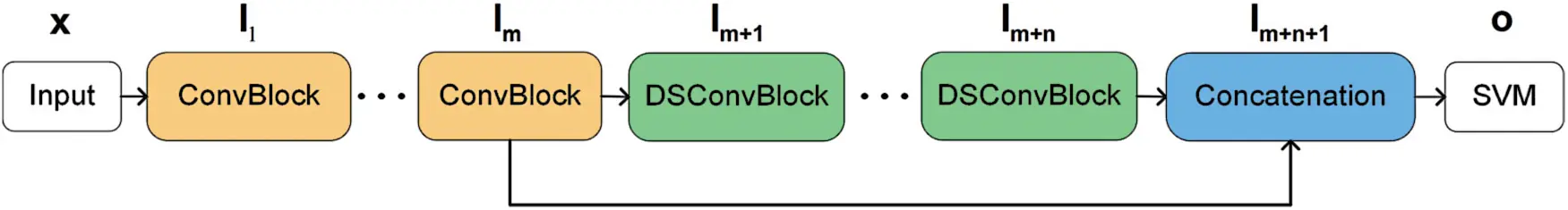Figure 1: A lightweight framework of cross-connected convolution kernel mapping support vector machines

The input of the framework is xRH×W×C, where H and W are the height and width of the image, respectively, and C is the number of channels of the image. The output of li(2im) is the output of the convolution module, lj(m+1jm+n) is the output of the depthwise separable convolution module, lm+n+1 is obtained by concatenating lm and lm+n through a cross-layerconnection, o which corresponds to the classification result of the support vector machine. The output of each layer of the framework is as follows:

{l1=CB(x)li=CB(li1),2imlj=DSCB(lj1),m+1jm+nlm+n+1=(Im,Im+n)o=wlm+n+1+b(1)

where w and b are the weights and biases of the soft margin support vector machine layer. The convolution module is denoted by CB(), the depthwise separable convolution module is indicated by DSCB().

2.2 Description of ConvBlock and DSConvBlock

The standard convolution (Conv) as well as the depthwise separable convolution (DSConv) are shown in Fig. 2. As can be seen from it, DSConv includes deepwise convolution, pointwise convolution and activation function, in which deep convolution is a grouped convolution with g groups, and the number of parameters is only 1/g of normal convolution, while pointwise convolution integrates the results of these g groups, so the number of parameters of DSConv is smaller than that of Conv. Both are computed as follows.

{Conv(x)=f(wx+b)DSConv(x)=f(w1(w2x+b2)+b1)(2)

where f is the ReLU activation function. w2 and b2 denote the learnable weights and bias of the deepwise convolution, respectively, and w1 and b1 denote the learnable weights and bias of the pointwise convolution, respectively. indicates convolution operation.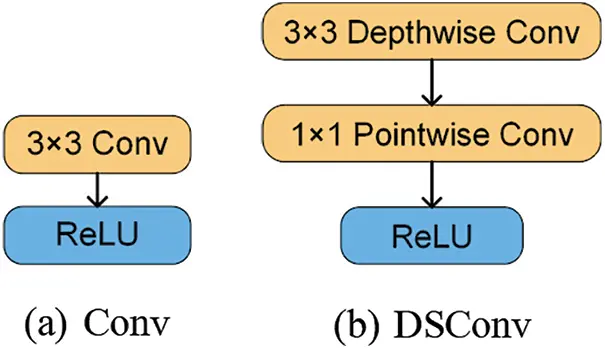Figure 2: Standard convolution and depthwise separable convolution

The structure of ConvBlock and DSConvBlock in the framework is shown in Fig. 3. The ConvBlock consists of several standard convolutions with a max pooling layer added at the end, while the DSConvBlock is a lightweight module consisting of several depthwise separable convolutions and a max pooling layer. The first part of the framework uses ConvBlock in order to extract the information of the image as completely as possible, and the second part is replaced by DSConvBlock in order to reduce the overall number of parameters of the framework. DSConvBlock replaces the DSConv with a DSConv that has fewer parameters than Conv to reduce the number of parameters. When both the standard convolution and the depthwise separable convolution are 2, the two modules are computed as follows.

{CB(x)=MaxPool(Conv2(Conv1(x)))DSCB(x)=MaxPool(DSConv2(DSConv1(x)))(3)

where MaxPool() denotes the max pooling operation, the 1st and 2nd standard convolution operations is symbolised by Conv1() and Conv2(), and the 1st and 2nd depthwise separable convolution operations is symbolised by DSConv1() and DSConv2(), respectively.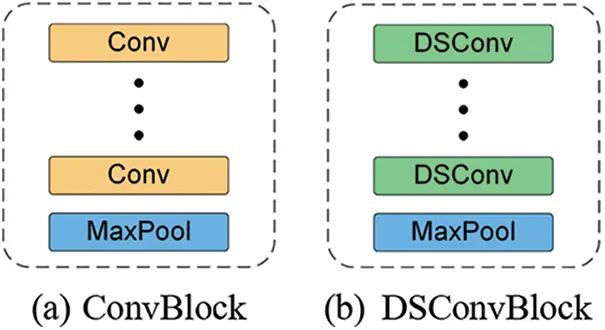Figure 3: Description of ConvBlock and DSConvBlock

2.3 Objective Function

The objective function of the LC-CKMSVM framework is shown in Eq. (4).

minw,b,ξ  12||w||2+Ck=1Nξk2s.t.  yk(wlm+n+1k+b)1ξk,  ξk0,1kN(4)

To simplify the above problem, Eq. (4) can be converted to an unconstrained form as shown in Eq. (5).

L=minw,b12||w||2+Ck=1Nmax(1yk(wlm+n+1k+b),0)2(5)

where N is the number of samples input, yk corresponds to the true value of the kth sample, lm+n+1k is the output of the kth sample at the m+n+1th module layer and also the high-dimensional feature of the kth sample after the feature extraction module, and the prediction value of the soft margin support vector machine for the kth sample is calculated by wlm+n+1k+b.

3  Experimental Results

In this section, experiments are conducted on two tasks, gender classification and character recognition, to verify the performance of the lightweight deep cross-connected convolutional kernel mapping support vector machine.

In this paper, mini batches stochastic gradient descent with momentum is used to update the model parameters. In all experiments, the number of training epochs is 90, the learning rate is set to 0.1, and every 30 rounds, the learning rate decreases to 1/10 of the original and the momentum is set to 0.9. The experiments are conducted on a server with processor model Intel® Xeon® E5-2643 and GPU model NVIDIA Tesla K40c, running 64-bit Windows 7. Python was used as the programming language and the experimental framework used was Pytorch .

3.1 Gender Classification

We evaluate the performance of the model using three datasets for gender classification, which are Labeled Faces in the Wild (LFW) , The Face Recognition Technology (FERET) , and The CelebFaces Attributes Dataset (CelebA) . Specifically, LFW is a publicly available dataset of 13,233 face images sourced from the Web. Each image in the dataset is labeled with the name of the person, and the images also contain information about different poses and expressions of the person, lighting changes. The dataset has been divided into a training set and a test set. The training set contains 8,125 male individuals and 2,367 female individuals, and the test set includes 2,053 male individuals and 598 female individuals. FERET contains 14,051 face images, and the face images are collected in a semi-monitored environment, with clean background. The training set contains 7,107 male images and 4,140 female images, and the test set contains 1,772 male images and 1,032 female images. CelebA is a large-scale face image dataset containing 202,599 face images collected from Internet. The dataset is divided into a training set containing 67,548 male individuals and 94,532 female individuals, and a test set containing 16,886 male individuals and 23,633 female individuals. The sample datasets used for the experiments are shown in Fig. 4. The figure shows the face images of both genders in each of the three datasets, with color images in the LFW dataset as well as the CelebA dataset and grayscale images in the FERET dataset. Besides, the images in both LFW and CelebA datasets have more complex background.Figure 4: Image examples derived from gender classification datasets

We compared our model with 5 other models, namely, RBFSVM, standard CNN, ConvMLP , CKMSVM, C-CKMSVM. In order to optimize the performance of the model on each dataset, the batch size is tuned in the range {16, 32, 64, 128, 256} in this paper, and the results are shown in Tab. 1. The parameter C of the RBFSVM model is used as 1 and the gamma value is used as 0.5.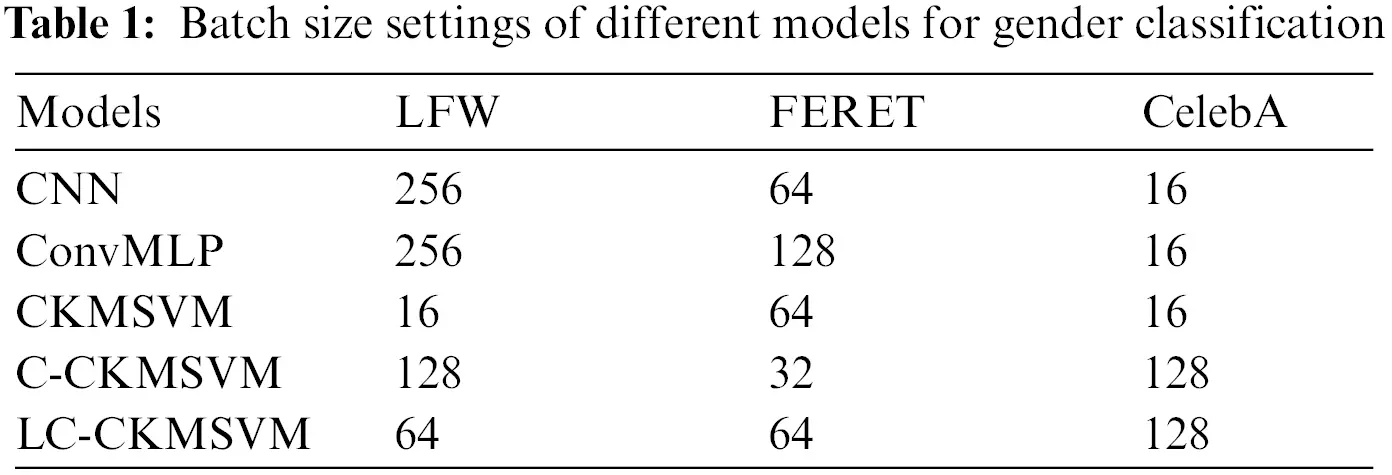The structure of the LC-CKMSVM model used in this paper is shown in Tab. 2. LC-CKMSVM fuses the output of ConvBlock2 with the output score of DSConvBlock, and then uses a soft margin support vector machine as a loss function for classification. ConvMLP uses the version of ConvMLP-S from the paper , in addition to reducing the hidden layer dimension.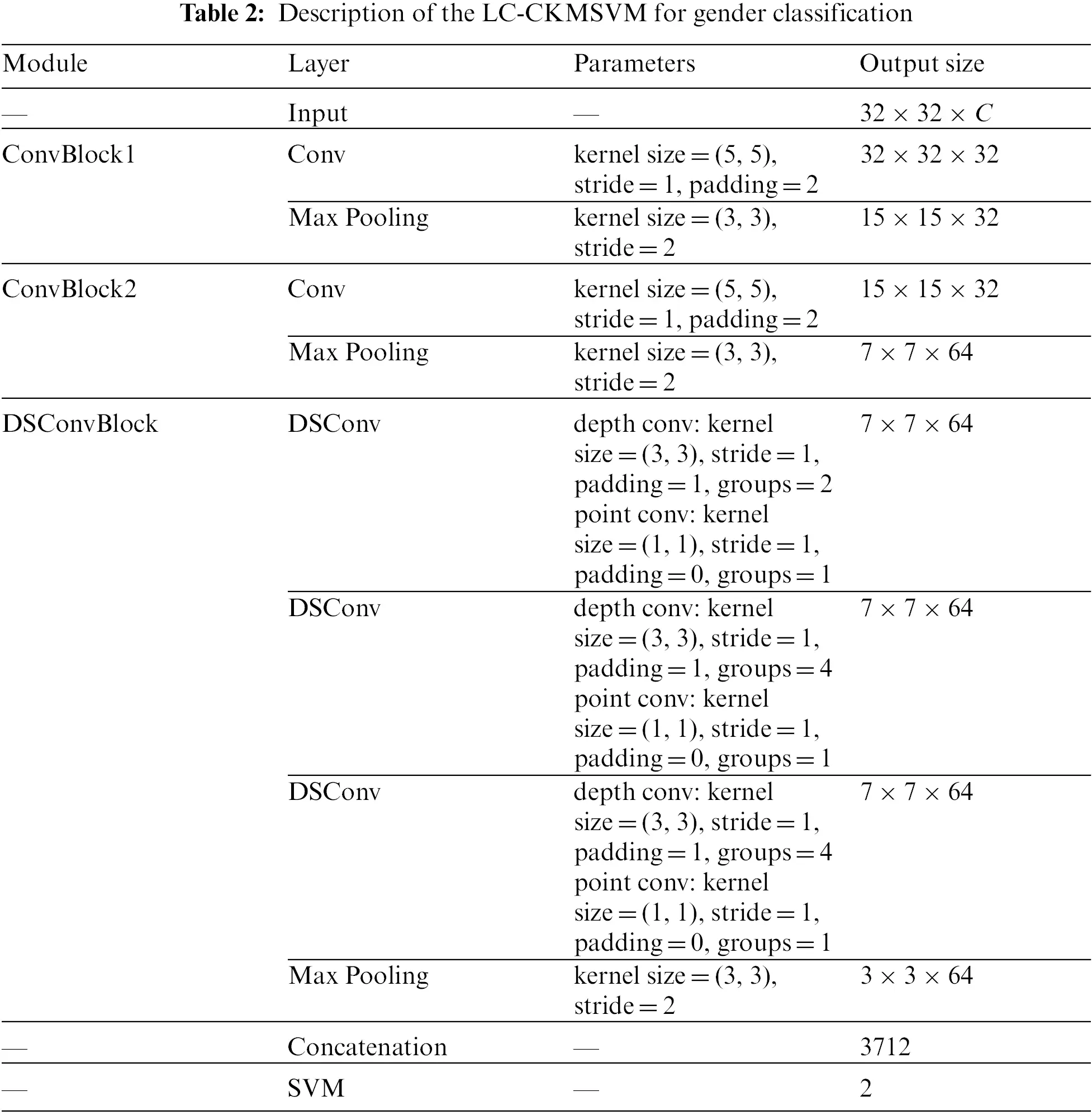The results of RBFSVM, CNN, ConvMLP, CKMSVM, C-CKMSVM, and LC-CKMSVM models on the testsets of three gender classification datasets, LFW, FERET, and CelebA, are shown in Tab. 3. The images in all three datasets were bilinearly interpolated so that the image size was maintained at 32×32, and the best test results are indicated in bold.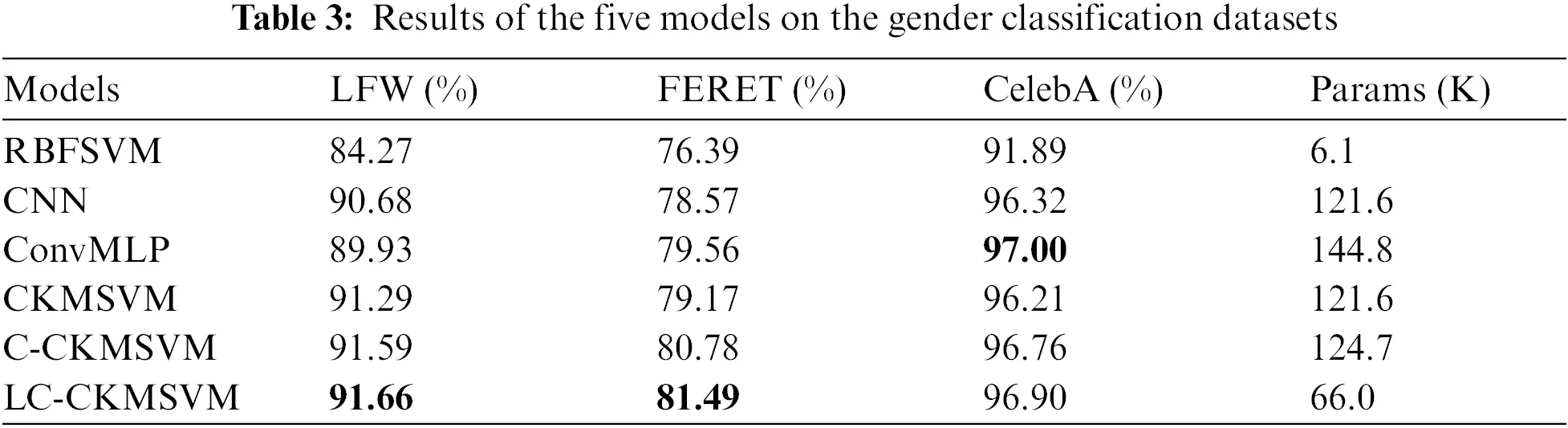From Tab. 3, it can be seen that:

(1)   On LFW, FERET, and CelebA datasets, the test accuracy of LC-CKMSVM is 91.66%, 81.49%, and 96.90%, respectively, and the test accuracy of RBFSVM is 84.27%, 76.39%, and 91.89%, respectively, and the test accuracy of LC-CKMSVM compared with RBFSVM model can improve 5.01%∼7.39%, indicating that the feature extraction module of this paper has better feature mapping ability compared with the RBF kernel.

(2)   On all the datasets, LC-CKMSVM is able to improve the test accuracy by 0.58%∼2.92% compared with the standard CNN model, which shows that the model proposed in this paper has better performance for gender classification. On the LFW and FERET datasets, the test accuracy of LC-CKMSVM was improved by 1.73% and 1.93% compared to ConvMLP, respectively. In addition, on the CelebA dataset, the test accuracy of LC-CKMSVM was slightly lower by 0.10% compared to ConvMLP, but the number of parameters was lower by 78.8 K compared to ConvMLP. Showing that LC-CKMSVM has better performance compared to ConvMLP.

(3)   On the three gender classification datasets, C-CKMSVM can improve the test accuracy by 0.30%∼1.61% compared with the CKMSVM model, showing that fusing features from different layers through cross-connected is effective for gender classification.

(4)   Compared with the C-CKMSVM model, LC-CKMSVM reduced the number of parameters from 124.7 to 69.1 K, while the test accuracy was able to improve 0.07%∼0.71% on LFW, FERET, and CelebA datasets. It indicates that LC-CKMSVM is able to ensure that the test accuracy can remain the same or even increase while the number of parameters is reduced on these three gender classification datasets.

(5)   In summary, LC-CKMSVM achieves better results and has better classification performance on gender classification tasks compared to RBFSVM, standard CNN, ConvMLP, CKMSVM, and C-CKMSVM.

3.2 Character Recognition

We experiment with three datasets for character recognition using EMNIST , KMNIST , and The Street View House Numbers (SVHN) , with models using RBFSVM, CNN, CKMSVM, and the lightweight C-CKMSVM. EMNIST is a handwritten character dataset, the experiments are conducted using EMNIST Letters, the total number of samples in the dataset is 145,600, and the number of categories is 26. KMNIST is a Japanese character dataset, the total number of samples in the dataset is 70,000, and the number of categories is 10. SVHN is a Google Street View house number image dataset with a training set of 73,257 samples, a test set of 26,032 samples, and a category number of 10. Partial examples of each dataset are shown in Fig. 5. The figure shows six images for each of the three datasets, where the images in EMNIST and KMNIST are grayscale images and the images in SVHN are color images.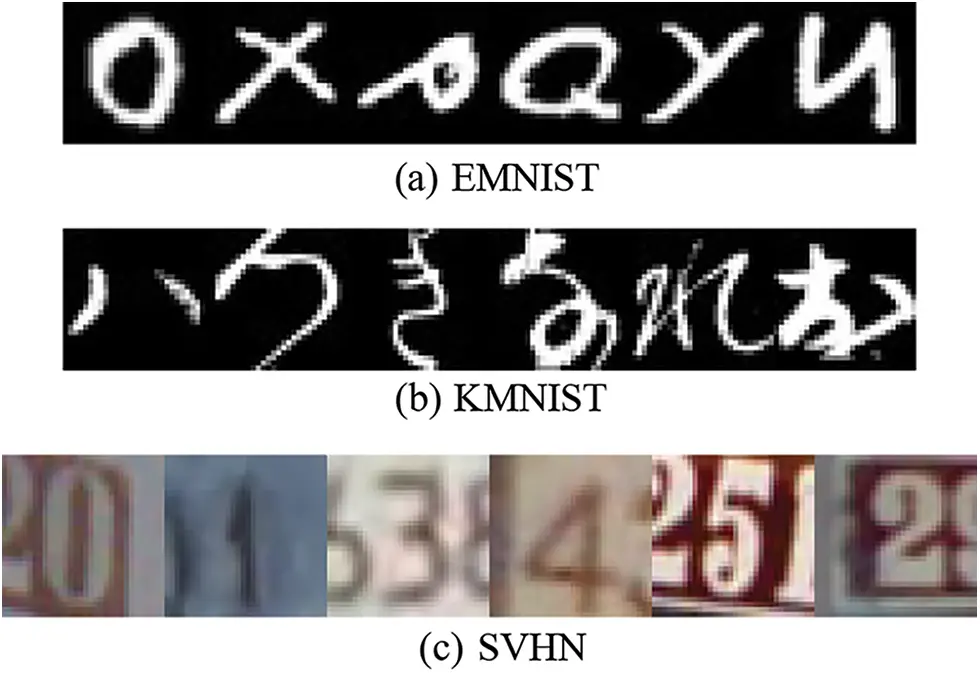Figure 5: Image examples derived from character recognition datasets

For these character recognition datasets, the batch size of the model is adjusted in this paper, and the final adjustment results are shown in Tab. 4. Among them, the parameter settings of RBFSVM are shown in Section 3.1.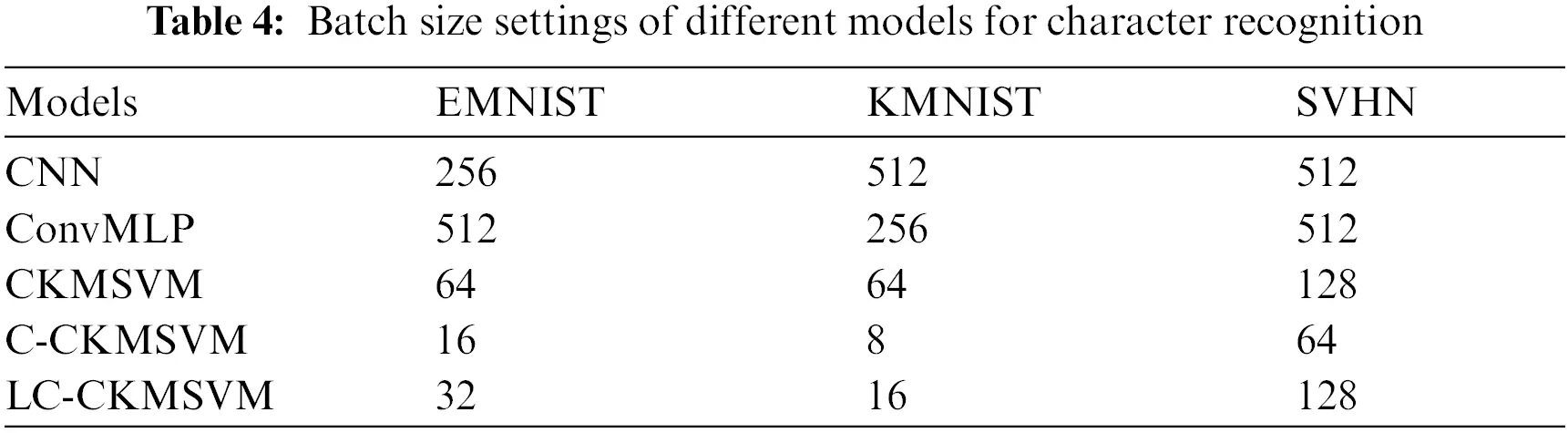The structure of LC-CKMSVM used for character recognition is shown in Tab. 5, where the convolution parameters are designed with reference to LeNet. The CKMSVM and ConvMLP processing is consistent with Section 3.1. The LC-CKMSVM fuses the output of ConvBlock with the output of DSConvBlock to obtain the final features of the feature extraction layer, and finally uses a soft margin support vector machine for character recognition.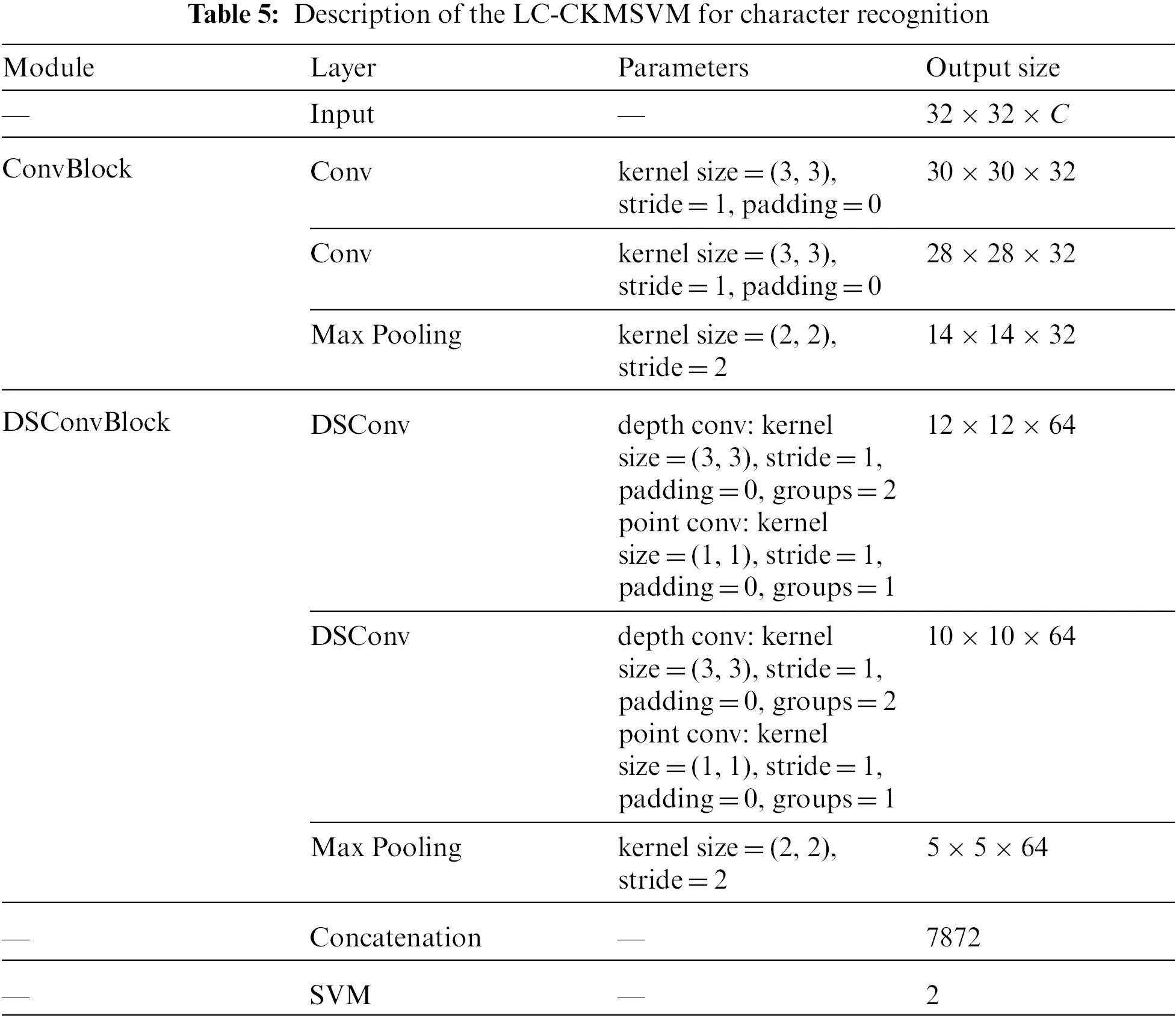The results of the five models on the character recognition task are shown in Tab. 6. From the table, it can be seen that:

(1)   the test accuracies of LC-CKMSVM on EMNIST, KMNIST, and SVHN datasets are 93.30%, 94.30%, and 88.80%, respectively, and the test accuracies of RBFSVM on these datasets are 70.05%, 72.73%, and 32.71%, respectively, LC-CKMSVM compared with RBFSVM improved by 23.25%, 21.57% and 56.09%, respectively, compared with RBFSVM.

(2)   LC-CKMSVM improves 0.14%, 0.76%, and 0.34%, respectively, compared to CNN on these three datasets. In addition, the test accuracy of LC-CKMSVM was improved by 0.20%, 0.59%, and 0.61% compared to ConvMLP on EMNIST, KMNIST, and SVHN datasets, respectively. LC-CKMSVM has a lower number of parameters compared to ConvMLP by 89.5 K.

(3)   LC-CKMSVM improved 0.34%, 0.67% and 0.94% compared to CKMSVM on these three datasets, respectively. Furthermore, the test accuracy of LC-CKMSVM on the three character recognition datasets were slightly reduced by 0.06%∼0.26% compared to C-CKMSVM, but the number of parameters was reduced from 81.3 to 55.3 K.

(4)   Overall, LC-CKMSVM was tested with higher accuracy in the character recognition task compared to RBFSVM, CNN, ConvMLP and CKMSVM, and with fewer number of parameters compared to C-CKMSVM.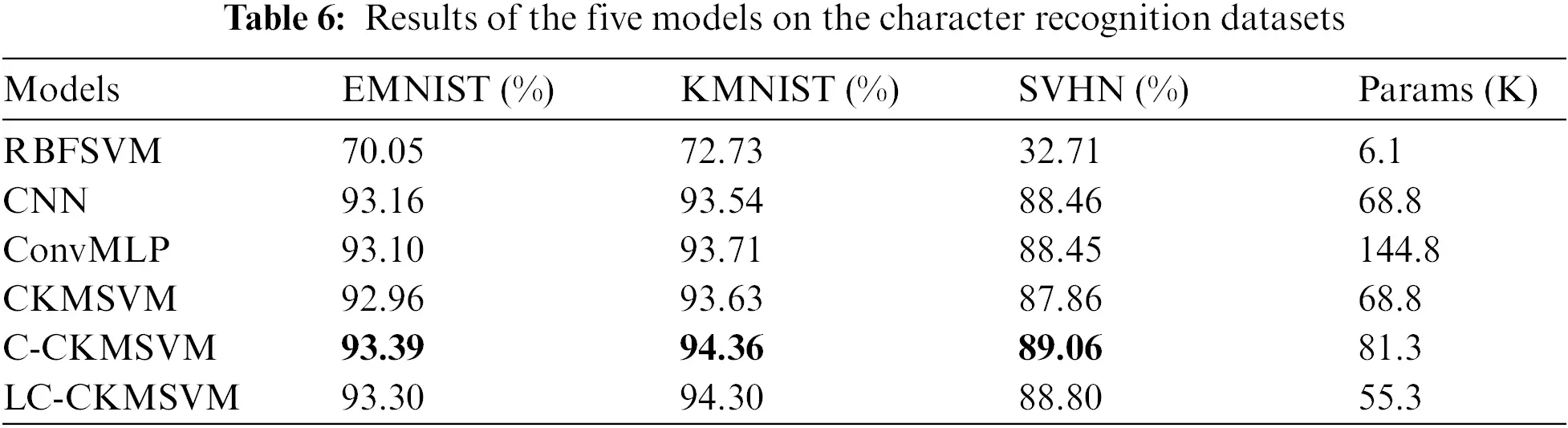4  Conclusions

In this paper, we propose a lightweight support vector machine framework for mapping deep cross-connected convolutional kernels. Firstly, the ordinary convolution in the low-level ConvBlock is replaced with a deepwise separable convolution to reduce the overall number of parameters of the model. Secondly using cross-layer connectivity, features between different modules are fused to enrich the final features to be classified. Finally, the mapped high-dimensional features are classified using a soft margin support vector machine. Experiments on three tasks with a total of 6 datasets show that the lightweight cross-connected convolutional kernel mapping support vector machine framework has better classification performance than RBFSVM, CNN, ConvMLP, and CKMSVM, and is able to reduce the number of parameters of the model to some extent compared to the standard deep cross-connected convolutional kernel mapping support vector machine. In future work, more feature fusion methods can be considered to enrich the features and further improve the accuracy of image classification.

Funding Statement: This work is supported by the National Natural Science Foundation of China (61806013, 61876010, 61906005, 62166002), General project of Science and Technology Plan of Beijing Municipal Education Commission (KM202110005028), Project of Interdisciplinary Research Institute of Beijing University of Technology (2021020101) and International Research Cooperation Seed Fund of Beijing University of Technology (2021A01).

Conflicts of Interest: The authors declare that they have no conflicts of interest to report regarding the present study.

## References

1. Y. Kessentini and T. Paquet, “Keyword spotting in handwritten documents based on a generic text line HMM and a SVM verification,” in Proc. ICDAR, Prouvé, Nancy, France, pp. 41–45, 2015.
2. M. Harmsen, B. Fischer, H. Schramm, T. Seidl and T. M. Deserno, “Support vector machine classification based on correlation prototypes applied to bone age assessment,” IEEE Journal of Biomedical & Health Informatics, vol. 17, no. 1, pp. 190–197, 2013.
3. K. Bagla and B. Bhushan, “A novel approach for face recognition using hybrid SIFT-SVM,” in Proc. ICPEICES, Delhi, India, Delhi Technological University, pp. 1–6, 2016.
4. C. Leslie, E. Eskin and W. S. Noble, “The spectrum kernel: A string kernel for SVM protein classification,” in Pacific Symp. on Biocomputing 2002, New York, World Scientific Publishing, vol. 7, pp. 564–575, 2002.
5. R. Bousseta, S. Tayeb, I. El Ouakouak, M. Gharbi, F. Regragui et al., “EEG efficient classification of imagined hand movement using RBF kernel SVM,” in Proc. SITA, Mohammedia, Morocco, Faculty of Sciences and Techniques of Mohammedia, pp. 1–6, 2016.
6. K. P. Bennett, M. Momma, M. J. Embrechts, “MARK: A boosting algorithm for heterogeneous kernel models,” in Proc. KDD-2002, Edmonton, Alberta, Canada, pp. 24–31, 2002.
7. S. Sonnenburg, G. Rätsch, C. Schäfer, “A general and efficient multiple kernel learning algorithm,” Advances in Neural Information Processing Systems, vol. 18, pp. 1–6, 2005.
8. Y. Li and T. Zhang, “Deep neural mapping support vector machines,” Neural Networks, vol. 93, pp. 185–194, 2017.
9. Z. Liu, H. Kan, T. Zhang and Y. Li, “Dukmsvm: A framework of deep uniform kernel mapping support vector machine for short text classification,” Applied Sciences, vol. 10, no. 7, pp. 2348, 2020.
10. K. He, X. Zhang, S. Ren, and J. Sun, “Deep residual learning for image recognition,” in Proc. CVPR, Caesar's Palace, Las Vegas, Nevada, pp. 770–778, 2016.
11. G. Huang, Z. Liu, V. Laurens and K. Q. Weinberger, “Densely connected convolutional networks,” in Proc. CVPR, Hawaii Convention Center, Honolulu, Hawaii, pp. 4700–4708, 2016.
12. T. Zhang, M. Waqas, Z. Liu, S. Tu, Z. Halim et al., “A fusing framework of shortcut convolutional neural networks,” Information Sciences, vol. 579, pp. 685–699, 2021.
13. Q. Zhang, M. Zhang, T. Chen, Z. Sun, Y. Ma et al., “Recent advances in convolutional neural network acceleration,” Neurocomputing, vol. 323, pp. 37–51, 2019.
14. A. G. Howard, M. Zhu, B. Chen, D. Kalenichenko, W. Wang et al., “Mobilenets: Efficient convolutional neural networks for mobile vision applications,” arXiv preprint arXiv:1704.04861, 2017.
15. K. He, X. Zhang, S. Ren and J. Sun, “Deep residual learning for image recognition,” in Proc. CVPR, Honolulu, Hawaii, Hawaii Convention Center, pp. 770–778, 2016.
16. M. Elhoushi, Z. Chen, F. Shafiq, Y. H. Tian and J. Y. Li, “Deepshift: Towards multiplication-less neural networks,” in Proc. CVPR, Online, pp. 2359–2368, 2021.
17. A. Paszke, S. Gross, F. Massa, A. Lerer and S. Chintala, “Pytorch: An imperative style, high-performance deep learning library,” Advances in Neural Information Processing Systems, vol. 32, pp. 8026–8037, 2019.
18. A. Jalal and U. Tariq, “The LFW-gender dataset,” in Asian Conf. on Computer Vision, Cham, Springer, vol. 2016, pp. 531–540, 2016.
19. J. Wolfshaar, M. F. Karaaba and M. A. Wiering, “Deep convolutional neural networks and support vector machines for gender recognition,” in IEEE Symp. Series on Computational Intelligence, Cape Town, South Africa IEEE, pp. 188–195, 2015.
20. Z. Liu, P. Luo, X. Wang and X. Tang, “Large-scale celebfaces attributes (celeba) dataset,” Retrieved August, vol. 15, pp. 11, 2018.
21. J. Li, A. Hassani, S. Walton and H. Shi, “Convmlp: Hierarchical convolutional mlps for vision,” arXiv preprint arXiv:2109.04454, 20
22. G. Cohen, S. Afshar, J. Tapson and A. V. Schaik, “EMNIST: Extending MNIST to handwritten letters,” in Proc. IJCNN, Anchorage, Alaska, US, pp. 2921–2926, 2017.
23. T. Clanuwat, M. Bober-Irizar, A. Kitamoto, A. Lamb, K. Yamamoto et al., “Deep learning for classical Japanese literature,” arXiv preprint arXiv:1812.01718, 2018.
24. Y. Netzer, T. Wang, A. Coates, A. Bissacco, B. Wu et al., “Reading digits in natural images with unsupervised feature learning,” in NIPS Workshop on Deep Learning and Unsupervised Feature Learning, Granada, Spain, 2011.This work is licensed under a Creative Commons Attribution 4.0 International License, which permits unrestricted use, distribution, and reproduction in any medium, provided the original work is properly cited.